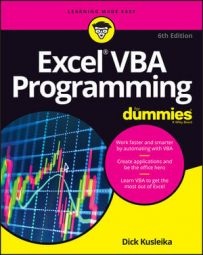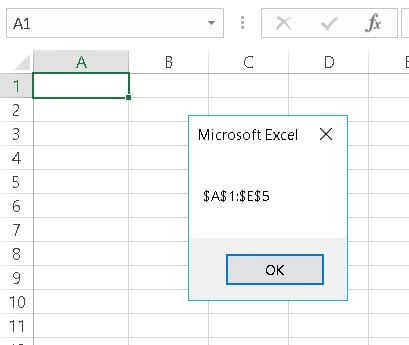##### Excel VBA Programming For DummiesA Range object has dozens of properties. You can write VBA programs nonstop for the next 12 months and never use them all. Here, you get a brief overview of some of the most commonly used Excel VBA Range properties. For complete details, consult the Help system in the VBE. (Check out these additional resources for help with Excel VBA.)

Some Excel VBA Range properties are read-only properties, which means that your code can look at their values but can't change them ("Look, but don't touch"). For example, every Excel VBA Range object has an Address property, which holds the range’s address. You can access this read-only property, but you can’t change it — which makes perfect sense when you think about it.

By the way, the examples that follow are typically statements rather than complete procedures. If you’d like to try any of these (and you should), create a Sub procedure to do so. Also, many of these VBA statements work properly only if a worksheet is the active sheet.

## Excel VBA: The Value property

The Value property represents the value contained in a cell. It’s a read-write property, so your VBA code can either read or change the value.

The following statement displays a message box that shows the value in cell A1 on Sheet1:

`MsgBox Worksheets("Sheet1").Range("A1").Value`
It stands to reason that you can read the Value property only for a single-cell Range object. For example, the following statement generates an error:
`MsgBox Worksheets("Sheet1").Range("A1:C3").Value`
You can, however, change the Value property for a range of any size. The following statement enters the number 123 into each cell in a range:
`Worksheets("Sheet1").Range("A1:C3").Value = 123`

Value is the default property for an Excel VBA Range object. In other words, if you omit a property for a Range, Excel uses its Value property. The following statements both enter a value of 75 into cell A1 of the active worksheet:

```Range("A1").Value = 75
Range("A1") = 75```

## Excel VBA: The Text property

The Text property returns a string that represents the text as it's displayed in a cell — the formatted value. The Text property is read-only. Suppose that cell A1 contains the value 12.3 and is formatted to display two decimals and a dollar sign (\$12.30). The following statement displays a message box containing \$12.30:
`MsgBox Worksheets("Sheet1").Range("A1").Text`
But the next statement displays a message box containing 12.3:
`MsgBox Worksheets("Sheet1").Range("A1").Value`
If the cell contains a formula, the Text property returns the result of the formula. If a cell contains text, the Text property and the Value property always return the same thing, because text (unlike a number) can't be formatted to display differently.

## Excel VBA: The Count property

The Count property returns the number of cells in a range. It counts all cells, not just the nonblank cells. Count is a read-only property, just as you would expect. The following statement accesses a range’s Count property and displays the result (9) in a message box:
`MsgBox Range("A1:C3").Count`

## Excel VBA: The Column and Row properties

The Column property returns the column number of a single-cell range. Its sidekick, the Row property, returns the row number of a single-cell range. Both are read-only properties. For example, the following statement displays 6 because cell F3 is in the sixth column:
`MsgBox Sheets("Sheet1").Range("F3").Column`
The next expression displays 3 because cell F3 is in the third row:
`MsgBox Sheets("Sheet1").Range("F3").Row`

If the Excel VBA Range object consists of more than one cell, the Column property returns the column number of the first column in the range, and the Row property returns the row number of the first row in the range.

Don’t confuse the Column and Row properties with the Columns and Rows properties. The Column and Row properties return a single value. The Columns and Rows properties, on the other hand, return a Range object. What a difference an “s” makes.

## Excel VBA: The Address property

Address, a read-only property, displays the cell address for a Range object as an absolute reference (a dollar sign before the column letter and before the row number). The following statement displays the message box shown below:
`MsgBox Range(Cells(1, 1), Cells(5, 5)).Address`This message box displays the Address property of a 5-by-5 range.

## Excel VBA: The HasFormula property

The HasFormula property (which is read-only) returns True if the single-cell range contains a formula. It returns False if the cell contains something other than a formula (or is empty). If the range consists of more than one cell, VBA returns True only if all cells in the range contain a formula or False if all cells in the range don’t have a formula. The property returns Null if the range contains a mixture of formulas and nonformulas. Null is kind of a no-man's land: The answer is neither True nor False, and any cell in the range may or may not have a formula.

You need to be careful when you work with properties that can return Null. More specifically, the only data type that can deal with Null is Variant.

For example, assume that cell A1 contains a value and cell A2 contains a formula. The following statements generate an error because the range doesn’t consist of all formulas or all nonformulas:
```Dim FormulaTest As Boolean
FormulaTest = Range("A1:A2").HasFormula```
The Boolean data type can handle only True or False. Null causes Excel to complain and display an error message. To fix this type of situation, the best thing to do is make sure that the FormulaTest variable is declared as a Variant rather than as a Boolean. The following example uses VBA’s handy TypeName function (along with an If-Then-Else construct) to determine the data type of the FormulaTest variable. If the range has a mixture of formulas and nonformulas, the message box displays Mixed! Otherwise, it displays True or False.
```Sub CheckForFormulas()
Dim FormulaTest As Variant
FormulaTest = Range("A1:A2").HasFormula
If TypeName(FormulaTest) = "Null" Then
MsgBox "Mixed!"
Else
MsgBox FormulaTest
End If
End Sub```

## Excel VBA: The Font property

A property can return an object. The Font property of an Excel VBA Range object is another example of that concept at work. The Font property returns a Font object.

A Font object, as you may expect, has many accessible properties. To change some aspect of a range’s font, you must first access the range’s Font object and then manipulate the properties of that object. This may be confusing, but perhaps this example will help.

The following statement uses the Font property of the Range object to return a Font object. Then the Bold property of the Font object is set to True. In plain English, this statement makes the cell display in boldface:

`Range("A1").Font.Bold = True`
Truth is, you don't really need to know that you're working with a special Font object that's contained in an Excel VBA Range object. As long as you use the proper syntax, it works just fine. Often, recording your actions with the macro recorder tells you everything you need to know about the proper syntax.

## Excel VBA: The Interior property

Here’s yet another example of a property that returns an object. A Range object’s Interior property returns an Interior object (strange name, but that’s what it’s called). This type of object referencing works the same way as the Font property.

For example, the following statement changes the Color property of the Interior object contained in the Range object:

`Range("A1").Interior.Color = 8421504`
In other words, this statement changes the cell’s background to middle gray. What's that? You didn't know that 8421504 is middle gray? For some insights into Excel's wonderful world of color, see the nearby sidebar “A quick-and-dirty color primer.”

## Excel VBA: The Formula property

The Formula property represents the formula in a cell. This is a read-write property, so you can access it to either view the formula in a cell or insert a formula into a cell. For example, the following statement enters a SUM formula into cell A13:
`Range("A13").Formula = "=SUM(A1:A12)"`
Notice that the formula is a text string and is enclosed in quotation marks. Also notice that the formula begins with an equal sign, as all formulas do.

If the formula itself contains quotation marks, things get a bit tricky. Say that you want to insert this formula by using VBA:

`=SUM(A1:A12)&" Stores"`
This formula displays a value followed by the word Stores. To make this formula acceptable, you need to replace every quotation mark in the formula with two quotation marks. Otherwise, VBA gets confused and claims that there's a syntax error (because there is!). So here's a statement that enters a formula that contains quotes:
`Range("A13").Formula = "=SUM(A1:A12)&"" Stores"""`
By the way, you can access a cell’s Formula property even if the cell doesn't have a formula. If a cell has no formula, the Formula property returns the same as its Value property.

If you need to know whether a cell has a formula, use the HasFormula property.

Be aware that VBA “speaks” U.S. English. This means that to put a formula in a cell, you must use the U.S. syntax. If you use a non-English version of Excel, read up on the FormulaLocal property in the Help system.

## Excel VBA: The NumberFormat property

The NumberFormat property represents the number format (expressed as a text string) of the Range object. This is a read-write property, so your VBA code can either examine the number format or change it. The following statement changes the number format of column A to a percentage with two decimal places:
`Columns("A:A").NumberFormat = "0.00%"`
Follow these steps to see a list of other number formats (better yet, turn on the macro recorder while you do this):
1. Activate a worksheet.
2. Press Ctrl+1 to access the Format Cells dialog box.
3. Click the Number tab.
4. Select the Custom category to view and apply some additional number format strings.